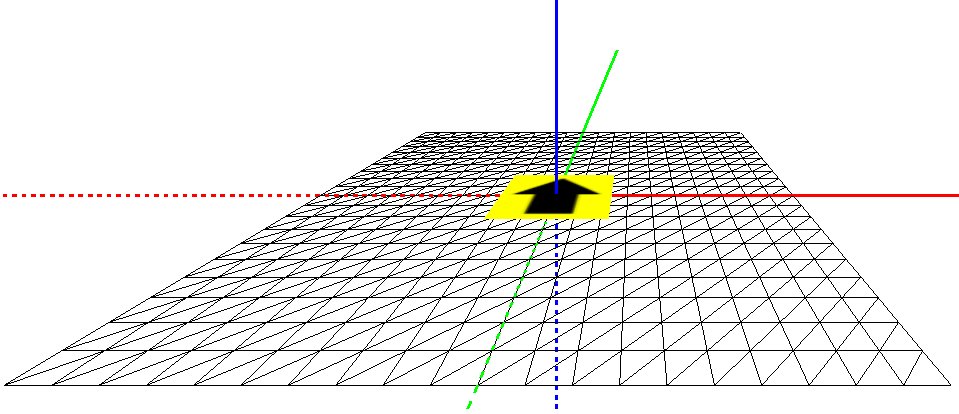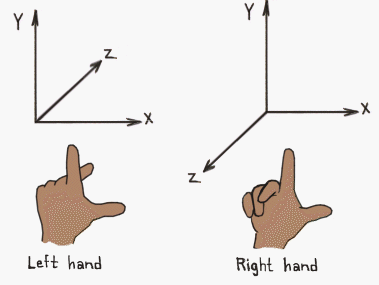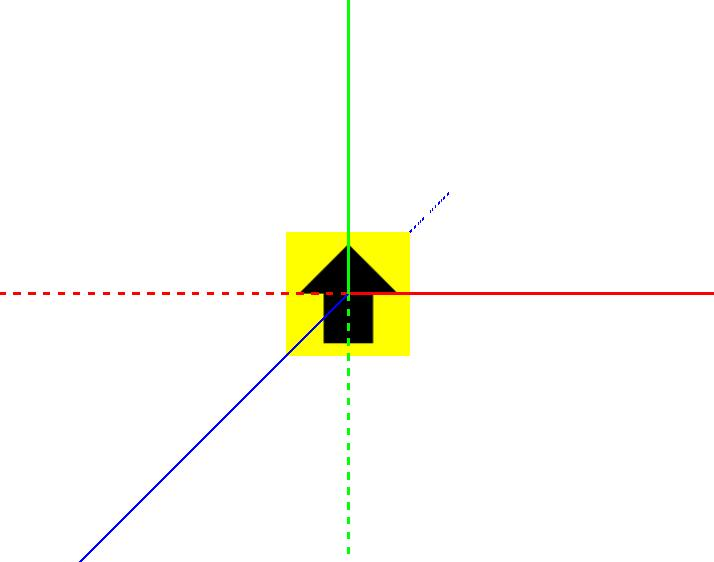April 17, 2015

# Threejs rotations

#### Daniel

Front-end developerIn this post we create a first person 3D setting, and we use rotations to accomplish this.

In Threejs you create a scene like so:

`` let scene = new THREE.Scene();``

This is the root scene; all other 3D objects have to be added to this root scene to make them visible:

``````  let obj3D = new THREE.Object3D(); // create a 3D object

The coordinate system of Threejs is a right handed system, which means that the positive z-axis is pointing towards you:In the picture below you see a Threejs scene. The red line is the x-axis, the green line the y-axis and the blue line the z-axis. A dotted line indicates a negative axis.

The black arrow in the yellow square is an instance of `THREE.PlaneBufferGeometry` which is a subclass of `THREE.Object3D`, the basic 3D object in Threejs.

On this PlaneBufferGeometry a texture of an arrow has been mapped. The PlaneBufferGeometry has been added to the root scene without any rotation or translation.What we can learn from this picture, is that a 3D object without translation and rotation gets added to the origin of the scene.

And as far as rotation is concerned: a 3D object that has no rotation on any of the three axes stands perpendicular to our line of sight and has its upside in the direction of the positive y-axis.

### Creating a floor

If we want to create a floor, or a ground for our 3D scene we have to rotate a plane -90° over the x-axis, play the following video to see how that works out:

If you rotate a 3D object in Threejs you only change its rotation in relation to the root scene: its own coordinate system is not affected. In the video above the positive y-axis of the PlaneBufferGeometry gets aligned with negative z-axis of the root scene.

What we could do as well, is to apply the rotation to the root scene as a whole; in that case the axes of the floor and the root scene stay aligned with each other:

Both solutions are equally valid, but there is one caveat: if you choose to rotate the root scene, please make sure that you do not add the camera to the scene because that would cancel out the rotations, see this post.

### Moving over a floor

Now lets create a proper floor and add the arrow object to the floor:Next we want to move the arrow object one unit into the direction the arrow head is pointing. We use trigonometry to calculate the fraction of the unit the arrow object has to move over the x-axis and the fraction of the unit the arrow object has to move over the y-axis based on its rotation over the z-axis:

``````  arrow.position.x += unit * Math.cos(arrow.rotation.z);
arrow.position.y += unit * Math.sin(arrow.rotation.z);``````

Because the z-rotation of the arrow object is 0° this boils down to:

``````  Math.cos(0) = 1;
Math.sin(0) = 0;

arrow.position.x += unit;
arrow.position.y += 0;``````

The translation of the arrow object on the x-axis is 1 unit, and the translation on the y-axis is 0, which means that the arrow object is moving over the red line (the x-axis) to the right instead of over the green line (the y-axis) away from us.

This is rather counter intuitive, and it is the result of the fact that in Threejs the top of a 0° rotated 3D object is in the direction of the positive y-axis, which is a very understandable decision because the y-axis is usually the vertical/upright axis.

We can fix this by rotating the floor or the root scene 90° over the z-axis. Lets move the root scene so the axes of the floor stay aligned with the axes of the root scene:

The arrow object is moving away from us but the head of the arrow points a the wrong direction. The rotation of the arrow object is still is 0°, but the texture on the arrow object (the PlaneBufferGeometry instance) makes us believe that the arrow object has a rotation of -90°.

We fix this in the arrow object itself by rotating the texture 90° which makes the direction of the arrow head consistent with the rotation of the PlaneBufferGeometry that it is applied to.

### Conclusion

If we rotate the root scene (or the floor) -90° over the x-axis, the y-axis becomes the 'away into the distance' axis, the natural axis that we want to move along when moving straight forward.

But because the natural angle of a straight forward movement is 0°, the x-axis actually is the most natural axis for moving forward, so we rotate the root scene (or the floor) 90° over the z-axis as well to swap the x and the y-axis.

Now we have created the ideal situation for a first person setting.

You can play yourself with the final result. Code is available at GitHub.

#### Daniel

daniel@tweedegolf.com

## Stay up-to-date

Stay up-to-date with our work and blog posts?